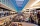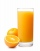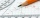# Fraction calculator

The calculator performs basic and advanced operations with fractions, expressions with fractions combined with integers, decimals, and mixed numbers. It also shows detailed step-by-step information about the fraction calculation procedure. Solve problems with two, three, or more fractions and numbers in one expression.

## Result:

### 43/5 + 71/2 = 121/10 = 12 1/10 = 12.1

Spelled result in words is one hundred twenty-one tenths (or twelve and one tenth).

### How do you solve fractions step by step?

1. Conversion a mixed number 4 3/5 to a improper fraction: 4 3/5 = 4 3/5 = 4 · 5 + 3/5 = 20 + 3/5 = 23/5

To find a new numerator:
a) Multiply the whole number 4 by the denominator 5. Whole number 4 equally 4 * 5/5 = 20/5
b) Add the answer from previous step 20 to the numerator 3. New numerator is 20 + 3 = 23
c) Write a previous answer (new numerator 23) over the denominator 5.

Four and three fifths is twenty-three fifths
2. Conversion a mixed number 7 1/2 to a improper fraction: 7 1/2 = 7 1/2 = 7 · 2 + 1/2 = 14 + 1/2 = 15/2

To find a new numerator:
a) Multiply the whole number 7 by the denominator 2. Whole number 7 equally 7 * 2/2 = 14/2
b) Add the answer from previous step 14 to the numerator 1. New numerator is 14 + 1 = 15
c) Write a previous answer (new numerator 15) over the denominator 2.

Seven and one half is fifteen halfs
3. Add: 23/5 + 15/2 = 23 · 2/5 · 2 + 15 · 5/2 · 5 = 46/10 + 75/10 = 46 + 75/10 = 121/10
For adding, subtracting, and comparing fractions, it is suitable to adjust both fractions to a common (equal, identical) denominator. The common denominator you can calculate as the least common multiple of both denominators - LCM(5, 2) = 10. In practice, it is enough to find the common denominator (not necessarily the lowest) by multiplying the denominators: 5 × 2 = 10. In the following intermediate step, the fraction result cannot be further simplified by canceling.
In other words - twenty-three fifths plus fifteen halfs = one hundred twenty-one tenths.

#### Rules for expressions with fractions:

Fractions - use the slash “/” between the numerator and denominator, i.e., for five-hundredths, enter 5/100. If you are using mixed numbers, be sure to leave a single space between the whole and fraction part.
The slash separates the numerator (number above a fraction line) and denominator (number below).

Mixed numerals (mixed fractions or mixed numbers) write as non-zero integer separated by one space and fraction i.e., 1 2/3 (having the same sign). An example of a negative mixed fraction: -5 1/2.
Because slash is both signs for fraction line and division, we recommended use colon (:) as the operator of division fractions i.e., 1/2 : 3.

Decimals (decimal numbers) enter with a decimal point . and they are automatically converted to fractions - i.e. 1.45.

The colon : and slash / is the symbol of division. Can be used to divide mixed numbers 1 2/3 : 4 3/8 or can be used for write complex fractions i.e. 1/2 : 1/3.
An asterisk * or × is the symbol for multiplication.
Plus + is addition, minus sign - is subtraction and ()[] is mathematical parentheses.
The exponentiation/power symbol is ^ - for example: (7/8-4/5)^2 = (7/8-4/5)2

#### Examples:

subtracting fractions: 2/3 - 1/2
multiplying fractions: 7/8 * 3/9
dividing Fractions: 1/2 : 3/4
exponentiation of fraction: 3/5^3
fractional exponents: 16 ^ 1/2
adding fractions and mixed numbers: 8/5 + 6 2/7
dividing integer and fraction: 5 ÷ 1/2
complex fractions: 5/8 : 2 2/3
decimal to fraction: 0.625
Fraction to Decimal: 1/4
Fraction to Percent: 1/8 %
comparing fractions: 1/4 2/3
multiplying a fraction by a whole number: 6 * 3/4
square root of a fraction: sqrt(1/16)
reducing or simplifying the fraction (simplification) - dividing the numerator and denominator of a fraction by the same non-zero number - equivalent fraction: 4/22
expression with brackets: 1/3 * (1/2 - 3 3/8)
compound fraction: 3/4 of 5/7
fractions multiple: 2/3 of 3/5
divide to find the quotient: 3/5 ÷ 2/3

The calculator follows well-known rules for order of operations. The most common mnemonics for remembering this order of operations are:
PEMDAS - Parentheses, Exponents, Multiplication, Division, Addition, Subtraction.
BEDMAS - Brackets, Exponents, Division, Multiplication, Addition, Subtraction
BODMAS - Brackets, Of or Order, Division, Multiplication, Addition, Subtraction.
GEMDAS - Grouping Symbols - brackets (){}, Exponents, Multiplication, Division, Addition, Subtraction.
Be careful, always do multiplication and division before addition and subtraction. Some operators (+ and -) and (* and /) has the same priority and then must evaluate from left to right.

## Fractions in word problems:

• Series and sequencesFind a fraction equivalent to the recurring decimal? 0.435643564356
• SavingsEva borrowed 1/3 of her savings to her brother, 1/2 of savings spent in the store and 7 euros left. How much did she save?
• Jo walksJo walks 3/4 of km to a friends home, 1/2 km to mall, and 2/3 km home. What total distance that joy covers?Mike buys flowers to plant around his trees. 3/8 of the flowers are red. 1/3 of the flowers are pink. The rest of the flowers are white. Find the fraction of flowers that are white.
• Sign of a expressionWhat is the sign of expression: minus 18 start fraction 9 divided by 17 end fraction plus left parenthesis minus 18 start fraction 9 divided by 17 end fraction right parenthesis?
• Lengths of the poolMiguel swam 6 lengths of the pool. Mat swam 3 times as far as Miguel. Lionel swam 1/3 as far as Miguel. How many lengths did Mat swim?To three-eighths of one third, we add five quarters of one half and multiply the sum by four. How much will we get?Write decimal number 8.638333333 as a fraction A/B in the basic form. Given decimal has infinite repeating figures.Patel squeezed oranges so that his family could have fresh-squeezed juice for breakfast. He squeezed 4/17 cups from the first orange, 3/10 cups from the second orange, StartFraction 9 over 20 EAdd this two mixed numbers: 1 5/6 + 2 2/11=The sum of two rational numbers is (-2). If one of them is 3/5, find the other.Calculate the sum of 1/5 of a right angle and 3/4 of a right angle and 3/4 of a straight angleNadia spent 1/4 of her money on a shirt and 2/5 of her money on new shoes. What fraction of Nadia´s money has been spent what fraction of her money is left?